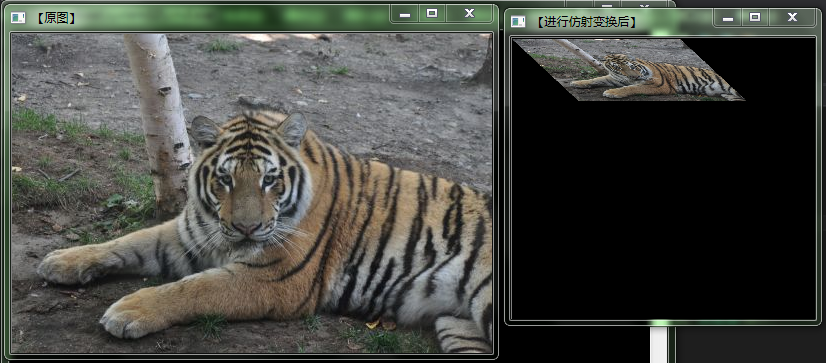## opencv3计算变换矩阵getAffineTransform函数和进行仿射变换warpAffine函数_轩落_翼的博客-程序员秘密

``````#include<opencv2/opencv.hpp>
#include<iostream>
#include<vector>

using namespace cv;
using namespace std;

int main()
{
imshow("【原图】", srcImage);

Mat dstImage;
dstImage.create(srcImage.size(), srcImage.type());
Mat MArray(2, 3, CV_32FC1);

输入源三角形的三个点的坐标，和输出三角形的三个点的坐标，建立对应关系
//Point2f srcPoint2f, dstPoint2f;
//srcPoint2f = Point2f(static_cast<float>(0), static_cast<float>(0));
//srcPoint2f = Point2f(static_cast<float>(srcImage.cols - 1), static_cast<float>(0));
//srcPoint2f = Point2f(static_cast<float>(0), static_cast<float>(srcImage.rows - 1));

//dstPoint2f = Point2f(static_cast<float>(0), static_cast<float>(srcImage.rows * 0.33));
//dstPoint2f = Point2f(static_cast<float>(srcImage.cols * 0.65), static_cast<float>(srcImage.rows * 0.35));
//dstPoint2f = Point2f(static_cast<float>(srcImage.cols * 0.15), static_cast<float>(srcImage.rows * 0.6));

//输入源三角形的三个点的坐标，和输出三角形的三个点的坐标，建立对应关系
Point2f srcPoint2f, dstPoint2f;
srcPoint2f = Point2f(static_cast<float>(0), static_cast<float>(0));
srcPoint2f = Point2f(static_cast<float>(0), static_cast<float>(3));
srcPoint2f = Point2f(static_cast<float>(3), static_cast<float>(0));

dstPoint2f = Point2f(static_cast<float>(0), static_cast<float>(3));
dstPoint2f = Point2f(static_cast<float>(3), static_cast<float>(5));
dstPoint2f = Point2f(static_cast<float>(5), static_cast<float>(3));

//用getAffineTransform函数建立对应关系
MArray = getAffineTransform(srcPoint2f, dstPoint2f);

warpAffine(srcImage, dstImage, MArray, Size(srcImage.cols * 3, srcImage.rows * 3), 1, 0, Scalar(0));

namedWindow("【进行仿射变换后】", 0);
imshow("【进行仿射变换后】", dstImage);

waitKey(0);

return 0;
}``````### stc15f104w模拟串口使用_weixin_30341735的博客-程序员秘密

stc15f104w单片机体积小，全8个引脚完全够一般的控制使用，最小系统也就是个电路滤波----加上一个47uf电容和一个103电容即可，但因为其是一个5V单片机，供电需要使用5V左右电源。 该款单片机视乎没有硬件串口，所以想要使用串口完成开发则应该使用软件模拟串口实现，其中P3.0为单片机RX,P3.1为单片机TX，下...

### 标注上下标的方法_iteye_3224的博客-程序员秘密

1）上标：编辑文字时，输入2^，然后选中2^，点a/b按键，即可。 （2）下标：编辑文字时，输入^2，然后选中^2，点a/b按键，即可。 （3）上下标：编辑文字时，输入2^2，然后选中2^2，点a/b按键，即可。 ...

### 随便推点

1、解码在上一篇博客《利用av_parser_parser2函数，解码h264文件》中，只能解码h264文件为yuv，不能直接从mp4、avi等多媒体文件中直接解码yuv，本文不仅可以解码h264文件到yuv，而且也支持直接从mp4中提取视频并解码为yuv，相比上一篇博客中的方法更通用一些。2、解码流程解码mp4文件比解码h264多了一个步骤：从视频流(stream)中复制参数到解码器上下文（AVCodecContext）中，这是一个必须的步骤，解码h264此步骤可以省略。3、代码v

### 少走弯路的十条忠告（转）_weixin_34356555的博客-程序员秘密

2019独角兽企业重金招聘Python工程师标准&gt;&gt;&gt; ...